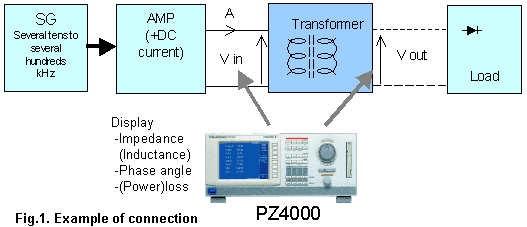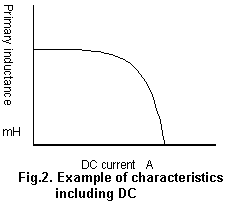# Evaluating Magnetic Components

PX8000 makes various evaluations of magnetic components as below;

Measurement of impedance (Inductance), phase angle and (power) loss.
PX8000 measures various parameters of impedance (Inductance), phase angle and (power) loss from high-frequency signals impressed on magnetic components, and displays such parameters.

Measurement of loss of the transformer
PX8000 with 4 input modules measures loss of primary and secondary output of the transformer.

Measurement of Inductance characteristics including DC current
Characteristic measurements, including DC current and inductance is measured by impressing a distorted AC signal of several tens to several hundreds kHz with changing DC current. PX8000 can measure both the high frequency distorted waveform and the DC.Performance required from power meter

• Measurement of impedance (Inductance), phase angle, and (power) loss
• High-precision measurement of distorted waveform signals including DC
• Calculation of efficiency and loss of input and output with multi input modules
Benefits for the user
• The PX8000 performs the functions of three instruments. Waveform observation, value display and harmonic measurements are performed in one instrument, saving the user test and evaluation time, cost and space for many instruments. The measuring results are highly reliable because the numeric calculations are based on the waveform data.
• You can input signals without using isolated amplifiers or current sensors. This reduces the error of such accessories.

### Related Products & Solutions

#### PX8000 - Precision Power Scope

• Transient/Start-up Waveform Capture & Power Analysis
• Harmonics, Cycle by cycle and FFT analysis

Top